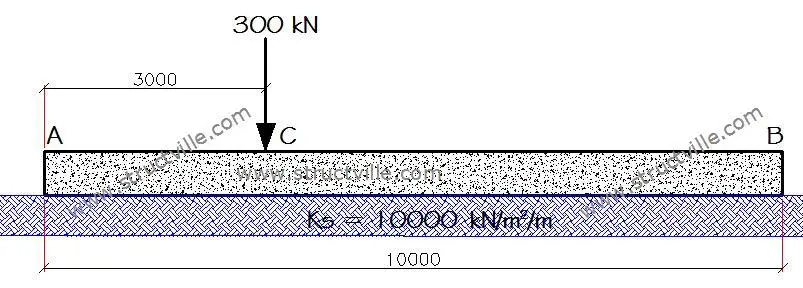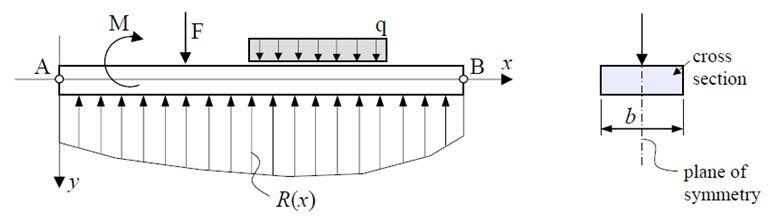# Analysis of Beams on Elastic FoundationIntroduction

When a beam lies on elastic foundation under the action of externally applied loads, the reaction forces of the foundation are proportional at every point to the deflection of the beam. This assumption was introduced first by Winkler in 1867. There are cases in which beams are supported on foundations which develop essentially continuous reactions that are proportional at each position along the beam to the deflection of the beam at that position. This is the reason for the name elastic foundation. There are many geotechnical engineering problems that can be idealized as beams on elastic foundations. This kind of modelling helps to understand the soil-structure interaction phenomenon and predict the contact pressure distribution and deformation within the medium (e.g. soil). The most common theory for a beam on elastic foundation modelling is the Winkler approach.Fig 1: Schematic representation of beam on elastic foundation

For instance, the beam shown in Fig. 1 will deflect due to the externally applied load, and produce continuously distributed reaction forces in the supporting medium. The intensity of these reaction forces at any point is proportional to the deflection of the beam y(x) at that point via the constant ks:

R(x) = ks.y(x)                (1)

Where kis the soil’s modulus of subgrade reaction which is the pressure per unit settlement of the soil (unit in kN/m2/m).

The reactions R(x) act vertically and opposing the deflection of the beam. Hence, where the deflection is acting downward there will be a compression in the supporting medium. Where the deflection happens to be upward in the supporting medium tension will be produced which is not possible (for soils).

If we assume that the beam under consideration has a constant cross section with constant width b which is supported by the foundation. A unit deflection of this beam will cause reaction equal to ks.b in the foundation, therefore the intensity of distributed reaction (per unit length of the beam) will be:

R(x) = b.ks.y(x) = k.y(x)                    (2)

where k = k0.b is the constant of the foundation, known as Winkler’s constant, which includes the effect of the width of the beam, and has unit of kN/m/m.

The general 4th order differential equation for beam on elastic foundation is given by equation (3);

EI(d4y)/dx4 + k.y = q                 (3)

The homogenous equation is given by;

EI(d4y)/dx4 + k.y = 0

(d4y)/dx4 + 4β4y = 0

Where β = ∜(k/4EI) = (k/EI)(1/4)

The general solution for the equation is available, which is given by;
y = eβx (C1sinβx + C2cosβx) + e-βx(C3sinβx + C4cosβx)

Warren and Richard (2002) published tables containing equations for analysing beams on elastic foundation subjected to different loads. The method described in the book has been employed to analyse a beam on elastic foundation and compare the results with Staad Pro software.

Solved Example
A 600mm x 400mm rectangular beam is resting on a homogenous soil of modulus of subgrade reaction ks = 10000 kN/m2/m. The beam is 10m long, and carrying a concentrated load of W =  300 kN from the left hand side. Neglecting the self weight of the beam, and assuming freely supported ends, obtain the bending moment at the point of the concentrated load. Take modulus of elasticity of concrete Ec = 21.7 x 106 kN/m2.

Solution
Second moment of area of concrete beam IB = (bh3)/12 = (0.4 × 0.63)/12 = 7.2 x 10-3 m4
Flexural rigidity of the beam EcIB = 21.7 × 106 × 7.2 × 10-3 = 156240 kN.m2
β = (bks/4EI)(1/4) = [(0.4 × 10000)/(4 × 156240)](1/4) = 0.2828
βl = 0.2828 × 10 = 2.828 m; β(l – a) = 0.282(10 – 3) = 1.979

Where a is the distance of the concentrated load from the left end of the beam.
Since βl < 6.0, we can use Table 8.5 of Roark’s Table for Stress and Strain (Warren and Richard, 2002).

For a beam with both ends free;
RA = 0; MA = 0

The equations for bending moment and shear force along the beam is as given below;

Mx = MAF1 + RA/2βF2 – yA2EIβ2F3 – θAEIβF4 – W/2βFa2
Vx = RAF1 – yA2EIβ3F2 – θAEIβ2F3 – MAβF4WFa1

Where;
θA (rotation at point A) = [W/(2EIβ2)] × [(C2Ca2 – 2C3Ca1)/C11]
yA (vertical deflection at point A) = [W/(2EIβ3)] × [(C4Ca1 – C3Ca2)/C11]

We can therefore compute the constants as follows;
C2 = coshβl.sinβl + sinhβl.cosβl = [cosh(2.828) × sin(2.828)] + [sinh(2.828) × cos(2.828)] = (8.485 × 0.3084) + (8.426 × –0.9512 ) = –5.398

C3 = sinhβl.sinβl = (8.426 × 0.3084) = 2.5985

C4 = coshβl.sinβl – sinhβl.cosβl = [cosh(2.828) × sin(2.828)] + [sinh(2.828) × cos(2.828)] = (8.485 × 0.3084) – (8.426 × –0.9512) = 10.631

Ca1 = coshβ(l – a).cosβ(l – a) = cosh(1.974) × cos(1.974) = 3.669 × -0.392 = -1.438

Ca2 = coshβ(l – a).sinβ(l – a) + sinhβ(l – a).cosβ(l – a) = [cosh(1.974) × sin(1.974)] + [sinh(1.974) × cos(1.974)] = (3.669 × 0.9198) + (3.530 × -0.392) = 1.9909

Ca3 = sinhβ(l – a).sinβ(l – a) = (3.530 × 0.9198) = 3.247

C11 = sinh2βl – sin2βl = 8.4262 – 0.30842 = 70.902

θA = W/(2EIβ2) × [(C2Ca2 – 2C3Ca1)/C11]
θA = [300/(2 × 156240 × 0.28282 ) × [(–5.398 × 1.9909) – (2 × 2.5985 × –1.438)/70.902] = (0.012 × –0.0462) = -0.0005544 radians

yA (vertical deformation at point A) = [W/(2EIβ3)] × [(C4Ca1 – C3Ca2)/C11]
y = [300/(2 × 156240 × 0.28283)] × [(10.631 × –1.438) –2.5985 × 1.9909)/70.902] = (0.0424 × –0.2885) = –0.01223 m = -12.23 mm

Bending moment at point C
Substituting the values of deflection and slope into the equation for bending moment (note that the first and second terms of the equation goes to zero since RA = MA = 0);

Mx = – yAEIβ2F3  – θAEIβF4 – W/2βFa2
Mx = – (–0.01223 × 2 × 156240 × 0.28282)F3 – (– 0.0005544 × 156240 × 0.2828)F4 – 300/(2 × 0.2828)Fa2

Mx = 305.638F3 + 24.495F4 – 530.410Fa2

Substituting F3, F4, and Fa2 (see Table 8.5, Warren and Richard, 2002) into the equation;

Mx = 305.638(sinhβx.sinβx) + 24.495(coshβx.sinβx – sinhβx.cosβx) – 530.410[coshβ(x –a).sin β(x –a) + sinh β(x –a).cos β(x –a)]

The bending moment under the concentrated load (point C);
x = 3m; (x – a) = 3 – 3 = 0

βx = 0.8484
Mx = 305.638[sinh(0.8484) × sin(0.8484)] + 24.495[cosh(0.8484) × sin(0.8484) – sinh(0.8484) × cos(0.8484)]

Mc = 305.638 (0.953 × 0.750) + 24.495(1.382 × 0.750 – 0.9539 × 0.6611) = 218.45 + 9.942 = 228.392 kN.m

Verification
This manual calculation has been verified using Staad Pro software. The steps adopted were as follows:

(1) Modelling
The 10m beam was modelled as a one dimensional line element connected by nodes at 1m length interval. This was to represent/attach soil springs at 1m interval.

(2) Section Properties
The beam was modelled using concrete of modulus of elasticity = 21.7 x 106 kN/m2, with dimensions of 600mm x 400mm.
(3) Support
Using elastic mat foundation option will not work since the support will not form a closed loop (analysing this way will give ‘colinear support error’). Therefore, the soil spring was modelled using ‘FIXED BUT’ support option. The support must be released for moment since this is the ideal scenario for the structure we are trying to model.
The soil modulus of subgrade reaction was multiplied with the width of the beam thus;
kb = 10000 kN/m2/m x 0.4m = 4000 kN/m2
Now, given that the nodes are spaced at 1m interval, the vertical spring constant was taken as 4000 kN/m. The general form of the foundation is given below;
(4) Analysis and Results
When analysed using static check option, the following results were obtained;
(a) Soil deformation
The vertical deflection at point A (node 1) was observed to be 12.195mm, against 12.23mm obtained using manual analysis.
(b) Bending Moment
The bending moment obtained at point C was 228.146 kNm which is comparable with 228.392 kNm obtained using manual calculations.
Therefore, it can be seen that both methods give approximately the same result.
References
Warren C.Y., Richard B. Y. (2002): Roark’s Formula for Stress and Strain (7th edition). McGraw Hill, USA

1.Mmabatho Lekalakala

Hi

May you kindly elaborate on the results received for soil deformation, especial from 8.5m to 10m?

Do the positive values not imply tension forces within the supporting soil medium?

Thanks and Regards,
ML

•Ubani Obinna

When a beam is resting on an elastic medium, it is assumed that it is not anchored to the soil. The physical interpretation is that there is a slight uplift of the beam at those points, thereby preventing contact with the soil. As this is undesirable, the designer can adopt some measures to ensure ‘no tension’ in the soil.

2.Hoshang Mustafa

Hi
I think a in Roark’s formula is the distance from left to the intermediate of the beam. So, a=10/2=5 and x-a=3-5